## Probability

Probability is the numerical measure of the chance of an outcome or event occurring. When all outcomes are equally likely to occur, the probability of the occurrence of a given outcome can be found by using the following formula:##### Example 1

Using the spinner shown in Figure 1, what is the probability of spinning a 6 in one spin?

Since there is only one 6 on the spinner out of ten numbers and all the numbers are equally spaced, the probability is.

##### Example 2

Again using the spinner shown in Figure 1, what is the probability of spinning either a 3 or a 5 in one spin?

Since there are two favorable outcomes out of ten possible outcomes, the probability isor.

When two events are independent of each other, you need to multiply to find the favorable and/or possible outcomes.

##### Example 3

What is the probability that both of the spinners shown in Figure 2 will stop on a 3 on the first spin?

Since the probability that the first spinner will stop on the number 3 isand the probability that the second spinner will stop on the number 3 is, and because each event is independent of the other, simply multiply.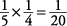##### Example 4

What is the probability that on two consecutive rolls of a die the numbers will be 2 and then 3? (A die has 6 sides numbered 1–6.)

Since the probability of getting a 2 on the first roll is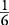and the probability of getting a 3 on the second roll is, and since the rolls are independent of each other, simply multiply.##### Example 5

What is the probability of tossing heads three consecutive times with a two‐sided fair coin?

Because each toss is independent and the probability is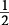for each toss, the probability is##### Example 6

What is the probability of rolling two dice in one toss so that they total 5?

Since there are six possible outcomes on each die, the total possible outcomes for two dice is

6 × 6 = 36

The favorable outcomes are (1 + 4), (4 +1), (2 + 3), and (3 + 2). These are all the ways of tossing a total of 5 on two dice. Thus, there are four favorable outcomes, which give the probability of throwing a total of five as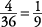##### Example 7

Three green marbles, two blue marbles, and five yellow marbles are placed in a jar. What is the probability of selecting at random a green marble on the first draw?

Since there are ten marbles (total possible outcomes) and three green marbles (favorable outcomes), the probability is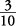.

##### Example 8

In a regular deck of 52 cards, what is the probability of drawing a heart on the first draw? (There are 13 hearts in a deck.)

Since there are 13 favorable outcomes out of 52 possible outcomes, the probability is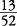or.

#### Arrangements

If there are a number of successive choices to make and the choices are independent of each other (order makes no difference), the total number of possible choices is the product of each of the choices at each stage.

##### Example 9

How many possible combinations of shirts and ties are there if there are five different color shirts and three different color ties?

To find the total number of possible combinations, simply multiply the number of shirts times the number of ties.

5 × 3 = 15

##### Example 10

A combination lock has three settings, each of which contains numbers from 0 to 9. How many different possible combinations exist on the lock?

Note that each setting is independent of the others; thus, because each has ten possible settings,

10 × 10 × 10 = 1,000

There are 1,000 possible combinations.

#### Permutations

If there are a number of successive choices to make and the choices are affected by the previous choice or choices (dependent upon order), then permutations are involved.

##### Example 11

How many ways can you arrange the letters S, T, O, P in a row?The product 4 × 3 × 2 × 1 can be written 4! (read 4 factorial or factorial 4). Thus, there are 24 different ways to arrange four different letters.

##### Example 12

How many different ways are there to arrange three jars in a row on a shelf?

Because the order of the items is affected by the previous choice(s), the number of different ways equals 3! or

3 × 2 × 1 = 6

There are six different ways to arrange the three jars.

##### Example 13

If, from among five people, three executives are to be selected, how many possible combinations of executives are there?

This is a more difficult type of arrangement involving permutations. Notice here that the order of selection makes no difference. The symbol used to denote this situation is

Cnr), which is read the number of combinations of n things taken r at a time. The formula used is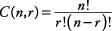Because n = 5 and r = 3 (five people taken three at a time), then the solution is as follows:Now solve.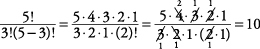If the problem involves very few possibilities, you may want to actually list the possible combinations.

##### Example 14

A coach is selecting a starting lineup for her basketball team. She must select from among nine players to get her starting lineup of five. How many possible starting lineups could she have?

Because n = 9 and r = 5 (nine players taken five at a time), the solution is as follows.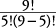##### Example 15

How many possible combinations of a, b, c, and d, taken two at a time, are there?

Since n = 4 and r = 2 (four letters taken two at a time), the solution is as follows:You may simply have listed the possible combinations as ab, ac, ad, bc, bd, and cd.